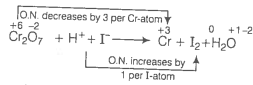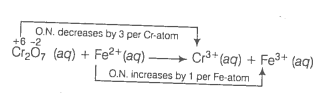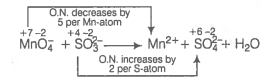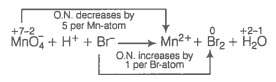26. Balance the following ionic equations.

(a) ${\mathrm{Cr}}_{2}{\mathrm{O}}_{7}^{2-}+{\mathrm{H}}^{+}+{\mathrm{I}}^{-}\to {\mathrm{Cr}}^{3+}+{\mathrm{I}}_{2}+{\mathrm{H}}_{2}\mathrm{O}$

(b) ${\mathrm{Cr}}_{2}{\mathrm{O}}_{7}^{2-}+{\mathrm{Fe}}^{2+}+{\mathrm{H}}^{+}\to {\mathrm{Cr}}^{3+}+{\mathrm{Fe}}^{3+}+{\mathrm{H}}_{2}\mathrm{O}$

(c) ${\mathrm{MnO}}_{4}^{-}+{\mathrm{SO}}_{3}^{2-}+{\mathrm{H}}^{+}\to {\mathrm{Mn}}^{2+}+{\mathrm{SO}}_{4}^{2-}+{\mathrm{H}}_{2}\mathrm{O}$

(d) ${\mathrm{MnO}}_{4}^{-}+{\mathrm{H}}^{+}+{\mathrm{Br}}^{-}\to {\mathrm{Mn}}^{2+}+{\mathrm{Br}}_{2}+{\mathrm{H}}_{2}\mathrm{O}$

(a) Write the O.N. of all atoms above their respective symbols.
O.N. decreases by, 3 per Cr-atomDivide the given equation into two half reactions
Reduction half reaction : ${\mathrm{Cr}}_{2}{\mathrm{O}}_{7}\to {\mathrm{Cr}}^{3+}$
Oxidation half reaction : ${\mathrm{I}}^{-}\to {\mathrm{I}}_{2}$
To balance reduction half reaction.
${\mathrm{Cr}}_{2}{\mathrm{O}}_{7}^{2-}+14{\mathrm{H}}^{+}+6{\mathrm{e}}^{-}\to 2{\mathrm{Cr}}^{3+}+7{\mathrm{H}}_{2}\mathrm{O}$
To balance oxidation half reaction.
$2{\mathrm{I}}^{-}\to {\mathrm{I}}_{2}+2{\mathrm{e}}^{-}$
To balance reaction by electrons gained and lost
$\frac{{\mathrm{Cr}}_{2}{\mathrm{O}}_{7}^{2-}+14{\mathrm{H}}^{+}+6{\mathrm{e}}^{-}\to 2{\mathrm{Cr}}^{3+}+7{\mathrm{H}}_{2}\mathrm{O}}{}$
$6{\mathrm{I}}^{-}\to 3{\mathrm{I}}_{2}+6{\mathrm{e}}^{-}{\mathrm{Cr}}_{2}{\mathrm{O}}_{7}^{2-}+14{\mathrm{H}}^{+}+6{\mathrm{I}}^{-}\to 2{\mathrm{Cr}}^{3+}+3{\mathrm{I}}_{2}+7{\mathrm{H}}_{2}\mathrm{O}$
This gives the final balanced ionic equations.
(b) Write the skeletal equation of the given reaction
${\mathrm{Cr}}_{2}{\mathrm{O}}_{7}^{2-}\left(\mathrm{aq}\right)+{\mathrm{Fe}}^{2+}\left(\mathrm{aq}\right)\to {\mathrm{Cr}}^{3+}\left(\mathrm{aq}\right)+{\mathrm{Fe}}^{3+}\left(\mathrm{aq}\right)$
Write the O.N. of all the elements above their respective symbols.Divide the given equation into two half reactions
Oxidation half reaction : ${\mathrm{Fe}}^{2+}\left(\mathrm{aq}\right)\to {\mathrm{Fe}}^{3+}\left(\mathrm{aq}\right)$
Reduction half reaction : ${\mathrm{Cr}}_{2}{\mathrm{O}}_{7}^{2-}\left(\mathrm{aq}\right)\to {\mathrm{Cr}}^{3+}\left(\mathrm{aq}\right)$
To balance oxidation half reaction
${\mathrm{Fe}}^{2+}\left(\mathrm{aq}\right)\to {\mathrm{Fe}}^{3+}\left(\mathrm{aq}\right)+{\mathrm{e}}^{-}$
To balance reduction half reaction
${\mathrm{Cr}}_{2}{\mathrm{O}}_{7}^{2-}\left(\mathrm{aq}\right)+6{\mathrm{e}}^{-}\to 2{\mathrm{Cr}}^{3+}\left(\mathrm{aq}\right)$
Balance charge by adding H+ ions.
${\mathrm{Cr}}_{2}{\mathrm{O}}_{7}^{2-}\left(\mathrm{aq}\right)+14{\mathrm{H}}^{+}+6{\mathrm{e}}^{-}\to 2{\mathrm{Cr}}^{3+}\left(\mathrm{aq}\right)$
Balance O atoms by adding H2O molecules
${\mathrm{Cr}}_{2}{\mathrm{O}}_{7}^{2-}\left(\mathrm{aq}\right)+14{\mathrm{H}}^{+}+6{\mathrm{e}}^{-}\to 2{\mathrm{Cr}}^{3+}\left(\mathrm{aq}\right)+7{\mathrm{H}}_{2}\mathrm{O}\left(\mathrm{l}\right)$
To balance the reaction
$\frac{6{\mathrm{Fe}}^{2+}\left(\mathrm{aq}\right)\to 6{\mathrm{Fe}}^{3+}\left(\mathrm{aq}\right)+6{\mathrm{e}}^{-}}{}$
${\mathrm{Cr}}_{2}{\mathrm{O}}_{7}^{2-}\left(\mathrm{aq}\right)+14{\mathrm{H}}^{+}\left(\mathrm{aq}\right)+6{\mathrm{e}}^{-}\to 2{\mathrm{Cr}}^{3+}\left(\mathrm{aq}\right)+7{\mathrm{H}}_{2}\mathrm{O}\left(\mathrm{l}\right){\mathrm{Cr}}_{2}{\mathrm{O}}_{7}^{2-}\left(\mathrm{aq}\right)+6{\mathrm{Fe}}^{2+}\left(\mathrm{aq}\right)+14{\mathrm{H}}^{+}\left(\mathrm{aq}\right)\to 2{\mathrm{Cr}}^{3+}\left(\mathrm{aq}\right)+7{\mathrm{H}}_{2}\mathrm{O}\left(\mathrm{l}\right)+6{\mathrm{Fe}}^{3+}\left(\mathrm{aq}\right)$
(c) Write the O.N. of all atoms above their respective symbols.Divide the skeleton equation into two half-reactions.
Reduction half reaction : ${\mathrm{MnO}}_{4}^{-}\to {\mathrm{Mn}}^{2+}$
Oxidation half reaction : ${\mathrm{SO}}_{3}^{2-}\to {\mathrm{SO}}_{4}^{2-}$
To balance reduction half reaction
${\mathrm{MnO}}_{4}^{-}+8{\mathrm{H}}^{+}+5{\mathrm{e}}^{-}\to {\mathrm{Mn}}^{2+}+4{\mathrm{H}}_{2}\mathrm{O}$
To balance oxidation half reaction
${\mathrm{SO}}_{3}^{2-}\to {\mathrm{SO}}_{4}^{2-}+2{\mathrm{e}}^{-}$
Balance charge by adding H+ ions.
${\mathrm{SO}}_{3}^{2-}\to {\mathrm{SO}}_{4}^{2-}+2{\mathrm{H}}^{+}+2{\mathrm{e}}^{-}$
${\mathrm{SO}}_{3}^{2-}+{\mathrm{H}}_{2}\mathrm{O}\to {\mathrm{SO}}_{4}^{2-}+2{\mathrm{H}}^{+}+2{\mathrm{e}}^{-}$
To balance the reaction
$\frac{2{\mathrm{MnO}}_{4}^{-}+16{\mathrm{H}}^{+}+10{\mathrm{e}}^{-}\to 2{\mathrm{Mn}}^{2+}+8{\mathrm{H}}_{2}\mathrm{O}}{}$
$5{\mathrm{SO}}_{3}^{2-}+5{\mathrm{H}}_{2}\mathrm{O}\to 5{\mathrm{SO}}_{4}^{2-}+10{\mathrm{H}}^{+}+10{\mathrm{e}}^{-}2{\mathrm{MnO}}_{4}^{-}+5{\mathrm{SO}}_{3}^{2-}+6{\mathrm{H}}^{+}\to 2{\mathrm{Mn}}^{2+}+5{\mathrm{SO}}_{4}^{2-}+3{\mathrm{H}}_{2}\mathrm{O}$
This represents the correct balance redox equation.
(d) Write the O.N. of all the atoms above their respective symbols.Divide skeleton equation into two half reactions
Reduction half reaction : ${\mathrm{MnO}}_{4}^{-}\to {\mathrm{Mn}}^{2+}$
Oxidation half reaction : ${\mathrm{Br}}^{-}+{\mathrm{Br}}_{2}$
To balance half reaction
${\mathrm{MnO}}_{4}^{-}+8{\mathrm{H}}^{+}+5{\mathrm{e}}^{-}\to {\mathrm{Mn}}^{2+}+4{\mathrm{H}}_{2}\mathrm{O}$
To balance oxidation half reaction
$2{\mathrm{Br}}^{-}\to {\mathrm{Br}}_{2}+2{\mathrm{e}}^{-}$
To balance the reaction
$\frac{2{\mathrm{MnO}}_{4}^{-}+16{\mathrm{H}}^{+}+10{\mathrm{e}}^{-}\to 2{\mathrm{Mn}}^{2+}+8{\mathrm{H}}_{2}\mathrm{O}}{}$
$10{\mathrm{Br}}^{-}\to 5{\mathrm{Br}}_{2}+10{\mathrm{e}}^{-}2{\mathrm{MnO}}_{4}^{-}+10{\mathrm{Br}}^{-}+16{\mathrm{H}}^{+}\to 2{\mathrm{Mn}}^{2+}+5{\mathrm{Br}}_{2}+8{\mathrm{H}}_{2}\mathrm{O}$
This represents the correct balance ionic equation.